Test: Dimensional Geometry - 6

# Test: Dimensional Geometry - 6

Test Description

## 20 Questions MCQ Test Topic-wise Tests & Solved Examples for IIT JAM Mathematics | Test: Dimensional Geometry - 6

Test: Dimensional Geometry - 6 for Mathematics 2023 is part of Topic-wise Tests & Solved Examples for IIT JAM Mathematics preparation. The Test: Dimensional Geometry - 6 questions and answers have been prepared according to the Mathematics exam syllabus.The Test: Dimensional Geometry - 6 MCQs are made for Mathematics 2023 Exam. Find important definitions, questions, notes, meanings, examples, exercises, MCQs and online tests for Test: Dimensional Geometry - 6 below.
Solutions of Test: Dimensional Geometry - 6 questions in English are available as part of our Topic-wise Tests & Solved Examples for IIT JAM Mathematics for Mathematics & Test: Dimensional Geometry - 6 solutions in Hindi for Topic-wise Tests & Solved Examples for IIT JAM Mathematics course. Download more important topics, notes, lectures and mock test series for Mathematics Exam by signing up for free. Attempt Test: Dimensional Geometry - 6 | 20 questions in 60 minutes | Mock test for Mathematics preparation | Free important questions MCQ to study Topic-wise Tests & Solved Examples for IIT JAM Mathematics for Mathematics Exam | Download free PDF with solutions
 1 Crore+ students have signed up on EduRev. Have you?
Test: Dimensional Geometry - 6 - Question 1

### The equation of the a straight lines bisecting the angles between the two straight lines ax2 + 2hxy + by2 = 0 is given by

Test: Dimensional Geometry - 6 - Question 2

### The polar equation of a straight line is given by ... where p is the length of the perpendicular from the origin on the line

Detailed Solution for Test: Dimensional Geometry - 6 - Question 2

Figure is self explanatory. From A OMP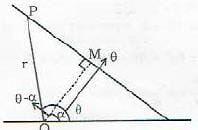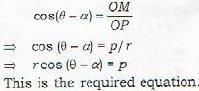Test: Dimensional Geometry - 6 - Question 3

### The polar equation of a line through two given points (r1, θ1) and (r2, θ2) is given by

Detailed Solution for Test: Dimensional Geometry - 6 - Question 3

A (r1, θ) and B(r2, θ2) arc two given points through them passes the straight line L. Let P(r, θ) be an arbitrary point on L.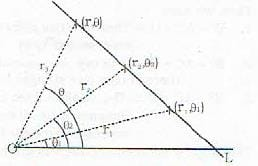Then the points P, A and B are collinear.
=>the area of the triangle formed by these three points is zero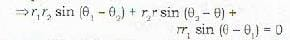Test: Dimensional Geometry - 6 - Question 4

If parallelograms are described on the sides of a given triangle treating them as diagonals of t he respective parallelograms having their sides parallel to two given straight lines, then the other diagonals of these parallelograms

Detailed Solution for Test: Dimensional Geometry - 6 - Question 4

The other diagonal will always meet in a point.

Test: Dimensional Geometry - 6 - Question 5

If the equations of a pair of opposite sides of a parallelogram are given by x2 - 7x + 6 = 0 and y2 -14y + 40 = 0, then the equation to one of it s diagonals is given by

Detailed Solution for Test: Dimensional Geometry - 6 - Question 5

x2- 7x + 6 - 0 => ( x - 1) ( x- 6) - 0
⇒ x= 1 and x = 6 are two opposite sides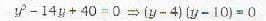=> y=4 and y = 10 are two opposite sides
Clearly the four vertices of the parallelogram are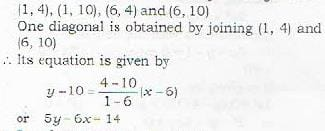Test: Dimensional Geometry - 6 - Question 6

The equation ax2 + by2 + c(x + y) = 0 represents a pair of straight lines if

Test: Dimensional Geometry - 6 - Question 7

The equation of the straight line which passes through the point (x' ,y') and is perpendicular to the straight line yy' = 2a[x + x']is given by

Detailed Solution for Test: Dimensional Geometry - 6 - Question 7

The given straight line is yy' = 2a(x + x') ...(i)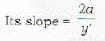the slope m of a straight line which ii perpendicular to (i) is given by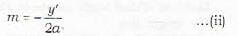Now the required equation passes through (x',y') and posseses thes slope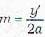∴ Its equation will be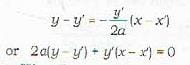Test: Dimensional Geometry - 6 - Question 8

The equation of the straight line passing through (x', y') and perpendicular to the straight line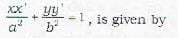Test: Dimensional Geometry - 6 - Question 9

The equations of straight lines passing through (x',y') and making an angle a with the given straight line y = mx + c, are given by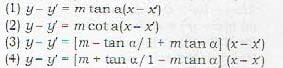Detailed Solution for Test: Dimensional Geometry - 6 - Question 9

Let m' be the slope of the straight line which makes an angle a with the line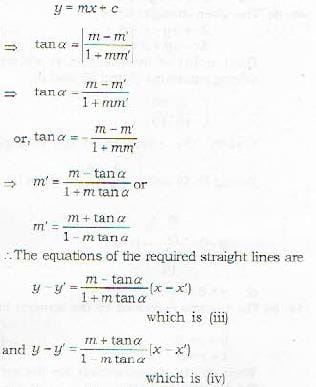Test: Dimensional Geometry - 6 - Question 10

The equation of one of the straight lines passing through the point (h, k) and making an angle tan-1 m with the. straight line y = mx + c is given ty

Detailed Solution for Test: Dimensional Geometry - 6 - Question 10

Proof: Let m' be the slope of the straight line which makes an angle tan-1 m with the straight line y = mx + c. Then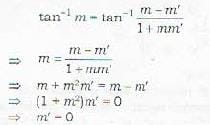∴ The straight line with slope m1 = 0 will be given by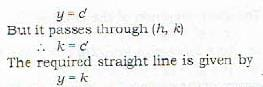Test: Dimensional Geometry - 6 - Question 11

The distance of the plane 6x -3y + 2z - 14 = 0 from the origin is

Detailed Solution for Test: Dimensional Geometry - 6 - Question 11

The length of the perpendicular from (x1, y1 ,z1) to the plane Ax+ By - Cz + D = 0
is given by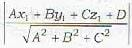∴ perpendicular distance d of the plane
6x-3y-2z -14=0
from the origin is given by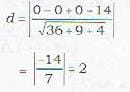Test: Dimensional Geometry - 6 - Question 12

In the equation of the plane given by ax + by + cz + d = 0: a. b, c denote the

Detailed Solution for Test: Dimensional Geometry - 6 - Question 12

Note that an equation of first degree in x, y, z of the form
Ax + By + Cz + D = 0 ...(i)
represents a plane
d.r.’s of the normal to (i) are A,B,C.
∴ d.e.'s of the normal to (i) are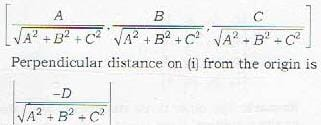Test: Dimensional Geometry - 6 - Question 13

The angle between the two planes is same as the angle between

Test: Dimensional Geometry - 6 - Question 14

The angle between the two planes ax + by + cz + d = 0 and a'x + b'y + c'z - a' = 0 is given by

Detailed Solution for Test: Dimensional Geometry - 6 - Question 14

Proof: The given planes are
ax + by + cz d = 0
and a'x + b'y + c'z + d' = 0 The d.r.’s of their normals are
a, b, c and a', b', c' repsectively.
∴ The d.c.’s of their normals are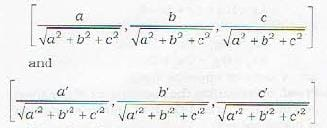Therefore if 0 is the required angle between the two planes (and hence between their normals), then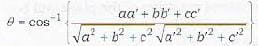That is why statements (a), (b) and (c) are not correct.

Test: Dimensional Geometry - 6 - Question 15

The two planes ax + by +by + cz + d = 0 and a'x + b'y + c'z + d = 0 are perpendicular if

Detailed Solution for Test: Dimensional Geometry - 6 - Question 15

Proof: The two planes are perpendicular if
θ = 90° or cos θ = 0
or aa' + bb' + cc' = 0
This is the condition of perpendicularity.
Remark : Condition of parallelism. Two planes are prallel if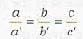Test: Dimensional Geometry - 6 - Question 16

The planes 2x - y + z = 15 and x + y + 2x = 3 are inclined at an angle of

Detailed Solution for Test: Dimensional Geometry - 6 - Question 16

The angle of inclination θ is given by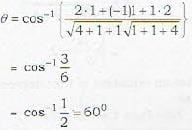Test: Dimensional Geometry - 6 - Question 17

Which one of the following is incorrect? The condition that the four points (x1, y1, z1), (x2, y2, z2), (x3, y3, z3) and (x4, y4, z4) are coplanar is

Detailed Solution for Test: Dimensional Geometry - 6 - Question 17

The condition that the four points (x1, y1, z1), (x2, y2, z2), (x3, y3, z3) and (x4, y4, z4) are coplanar is that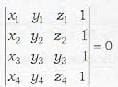Remark: The other three statements are same as he condition given above, (rows or columns in a determinant can be interchanged. Even number of changes do not change the value of the determinant. Odd number of changes make the value of the determinant as the negative of the original value)

Test: Dimensional Geometry - 6 - Question 18

Which of the following statements is incorrect? two points P(x1 , y1, z1) and Q(x2, y2, z2) lie on the same side of the plane ax - by + cz - d 0 if

Detailed Solution for Test: Dimensional Geometry - 6 - Question 18

Result: Two points P(x1 , y1, z1) and Q (x2 , y2, z2) lie: on the same side: or opposite sides of the: plane
Ax + By + Cz + D = 0 according as the quantities
Ax1 + By1 + Cz1 + D and
Ax2 + By2 + Cz2 + D are of same or opposite signs.

Test: Dimensional Geometry - 6 - Question 19

The two points (1, 1, 1) and (-3, 0, 1) from the plane 3x +4y - 12z + 13=0 are

Detailed Solution for Test: Dimensional Geometry - 6 - Question 19

Proof: Substituting the coordinates of the given points P( 1,1, 1) and Q (-3, 0, 1) in the equation of plane, we get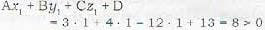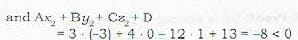Since they are of opposite signs, therefore the points lie on the opposite sides of the plane. Also the perpendicular distances p and q of P and Q are given by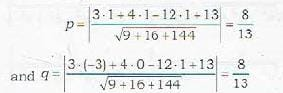Thus the points are equidistant from the plane and on the opposite sides of it.

Test: Dimensional Geometry - 6 - Question 20

If D > 0, then the length of the perpendicular from the origin on the plane Ax + By + Cz + D = 0 is

Detailed Solution for Test: Dimensional Geometry - 6 - Question 20

Length of perpendicular from origin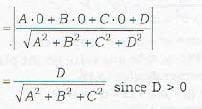## Topic-wise Tests & Solved Examples for IIT JAM Mathematics

27 docs|150 tests
 Use Code STAYHOME200 and get INR 200 additional OFF Use Coupon Code
Information about Test: Dimensional Geometry - 6 Page
In this test you can find the Exam questions for Test: Dimensional Geometry - 6 solved & explained in the simplest way possible. Besides giving Questions and answers for Test: Dimensional Geometry - 6, EduRev gives you an ample number of Online tests for practice

## Topic-wise Tests & Solved Examples for IIT JAM Mathematics

27 docs|150 tests